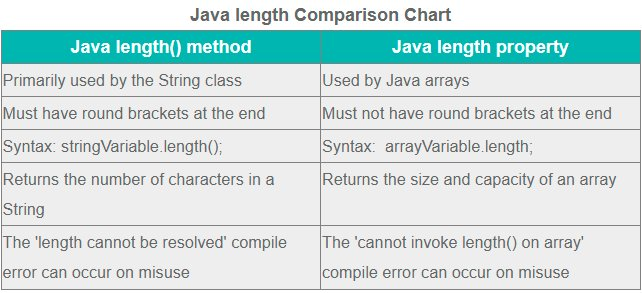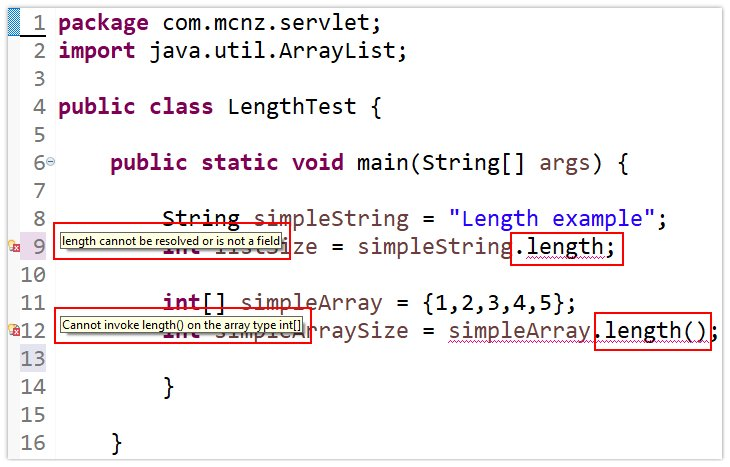# Java length vs length(): What's the difference?

## Java length vs length() explained

The key difference between Java’s length variable and Java’s length() method is that the Java length variable describes the size of an array, while Java’s length() method tells you how many characters a text String contains.

## The Java length method

To get information about an object in Java, you typically go through a public method.

To get the size of a String in Java, you call the String’s length() method. This tells you how many characters a String contains.

### A length() example for Java Strings

Here is a simple example of using the Java length() method to get the number of characters in a String:

```String simpleString = "Length example";
int listSize = simpleString.length();```

Notice the round brackets at the end of the Java length() method. This is one of the primary differences between the length() method and Java’s length property.

## The Java length property

To get the size of an array in Java, you access the array’s length property.

Notice how we have switched from talking about Java’s length() method to Java’s length property. This is a primary difference between the two uses of the word length in Java.

### A length example for Java arrays

Here is an example of using the Java length method to get the number of elements in an array:

```int[] simpleArray = {1,2,3,4,5};
int simpleArraySize = simpleArray.length;```

## Differences between Java length and length()

Here are the key differences between the two Java length constructs and how to use them in code:

1. The Java length property is used with the String class
2. The Java length method is used with the array class
3. The Java length property returns the size of an array
4. The Java length method returns the number of characters in a text String
5. The Java length method must have round brackets at the end
6. The Java length property throws a compile error if you add round brackets
7. The Java length method is a good example of data encapsulation
8. The Java length property has its roots in C and C++ programming

### Similarities between the Java length property and method

The biggest similarity between the two Java length constructs, be it for Strings or arrays, is that they help to determine the number of elements an object contains.

They key difference between Java length and length() constructs is that the method works with the String class, while the property works with arrays.

This subtle difference often confuses new developers who are just getting used to Java syntax. This is because:

• In Java, a method must have round brackets at the end of it: length()
• In Java, properties are not followed by brackets or braces: length

If you put round brackets at the end of a property, or left off a method name, this causes a Java compile time error. Sadly, the associated error message isn’t very helpful, as we see below.How to compare Java length versus length() for arrays and Strings.

## Java length compile time errors

If round brackets do not follow a Java length() method call, the following code error occurs:

`length cannot be resolved or is not a field`

If round brackets come after a Java length property, the following code error occurs:

```Cannot invoke length() on the array type int[]
```If Java length and length() syntax is mixed up, compile time errors occur.

## Why do arrays and Strings use the Java length differently?

Java values the encapsulation of data and properties, which means any information you access about an object should come through a public method.

The Java String’s length() method complies with this best practice. So why doesn’t an array?

The difference harks back to Java’s C -based heritage.

### C++, Java and the length of an array

The Java programming language was written in such a way that developers who program in C and C++ can more easily adopt this language.

Several programming constructs in Java map directly back to how C and C++ programs work. One of these constructs is Java’s main method. Another is the fact that you access the size of an array through the length property, not the length method.

The difference between Java length() versus length in Java can be confusing for new developers. It’s just a one-off rule about how arrays work differently from Strings that Java developers just have to accept.

You’ll get used to it eventually. Trust me.

Close